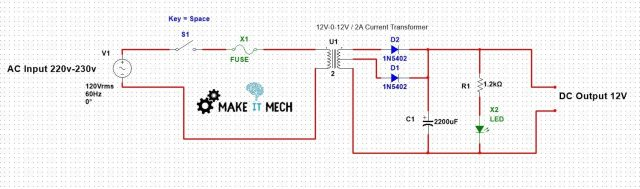">

# 220v AC to 12v DC Power Supply Step by Step Project

220v ac to 12v dc power supply is the most used and common circuit. There are so many applications of AC to DC converter Project. The 220v to 12v dc power supply is build to convert AC input to 12 volt DC output. The ac to dc converter project is useful for fixed DC applications like DC motors, pumps, Chargers and many other applications. Here we are going to discuss the what is a dc power supply and circuit for power supply for 12 volt output. The high current dc power supply is quite simple to test and build. This AC to DC converter Project of Power supply is a beginners level circuit for basic electronics projects. We are going to define how to make a 12v power supply. The circuit can be used with many useful applications as it draws 2-A Current. The ac to dc converter project is the best approach to make this easy and simple power supply project. This is a 12V DC adapter circuit.

## 220v Ac to 12v Dc Power Supply

### 1. Objective:

What is a dc power supply and how we can define our objective as how to make a 12v power supply. To convert 220V AC input into 12V DC output. The 12 Volt Fixed DC output is useful for many DC Controlled applications like DC motors, DC circuits, Pumps, Battery Chargers and many other useful applications.

### 2. Required Components:

 S.No Components List Quantity 1 2-Amp Transformer (12v-0-12v) CT 1 2 Diod (1N5402) – 3-Amp 2 3 Capacitor (2200uF) 1 4 Resistor (1.2kΩ)-0.5W 1 5 LED (RED) 1 6 Switch (SPST) 1 7 Fuse (1-Amp) 1

###3. Circuit for Power Supply:

The 220v ac to 12v dc power supply is easy and quite simple. The input voltage is 220 volts AC. This is also the AC to DC converter Project. Connect an AC wire plug at input and then a Switch and a fuse. The circuit is based upon a transformer. The transformer reduces the AC voltages from 220 to 12 volts. As we know that whenever we convert from ac to dc we need a rectifier circuit. The diodes are used to rectify the output. The output is 12 Volt DC.

###4. Working Principle AC to DC converter Project:

• The basic purpose of 220v ac to 12v dc power supply project is to make a 12V DC output voltages to run DC applications
• The fuse is used for the protection of the circuit.
• Connect the circuit input to Mains 220V AC 50/60 Hz.
• The 220 Volts Ac to 12 Volts DC current transformer is used to convert AC voltages to DC. The Current rating of transformer is 2-Amperes.
• The Diode Rectifier is used to rectify the AC input into 12V DC. The 1N5402 Diode is used to make a rectifier circuit.
• The use of capacitor here is to filter the output.
• The LED shows the rectified filtered output of 12 volts DC.
• Now you can connect any DC operated circuitry to 12-VDC output.

### 5. Output Results:

The filtered 12 volts DC output is generated. The simple transformer based power supply circuit output is 12 volts fixed DC. The output is not variable.it is fixed 12 volts dc voltages. These DC voltages can be utilized in any dc operated 12 volts AC to DC converter project. Like running a 12 volts motor, any circuitry that need 12 volts dc ,a dc fan, charger etc. This can be used as DC adapter. A 220v ac to 12v dc power supply project. There are so many

### 6. Applications of DC power supply.

You can get this project in PDF file as well. This is the most easiest and simplest 220v to 12v dc power supply. A quick learning tutorial about what is a dc power supply. This can be better a semester project as basic electronics AC to DC converter Project. The power supply project is the best showcase of basic electronics components.

We will discuss the variable DC power supply circuit project in coming posts. Subscribe to our YouTube channel for more tutorials and ideas. Stay motivated and always have faith on yourself….